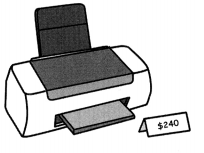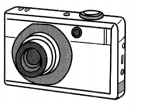# Math in Focus Grade 5 Chapter 10 Practice 4 Answer Key Real-World Problems: Percent

Practice the problems of Math in Focus Grade 5 Workbook Answer Key Chapter 10 Practice 4 Real-World Problems: Percent to score better marks in the exam.

## Math in Focus Grade 5 Chapter 10 Practice 4 Answer Key Real-World Problems: Percent

Question 1.
Jennifer bought a printer that cost $240. There was a 7% sales tax on the printer. a. How much sales tax did Jennifer pay?Answer:$16.8
Explanation:
240 x 7%
= (240  x 7)/100
=$16.8 b. How much did Jennifer pay for the printer with tax? Answer:$256.8
Expanation:
Printer cost $240 Tax paid 7% = &16.8 Tota amount paid =$240 + $16.8 =$256.8

Question 2.
A company invests $8,000 in an account that pays 6% interest per year. a. How much interest will the company earn at the end of 1 year? Answer:$480
Explanation:
$8000 x 6% = (8000 x 6)/100 = 480 b. How much money will the company have in the account at the end of 1 year? Answer:$8480
Explanation:
invested amount = $8000 interest 6% the company have in the account at the end of 1 year$8000 + $480 = 8480 Solve. Show your work. Question 3. The regular price of a digital camera was$250. Tyrone bought the digital camera at a discount of 40%. How much did Tyrone pay for the digital camera?$150 Tyrone pay for the digital camera. Explanation: camera price is$250
40% of 250 is
(250 x 40)/100 = 25 x 4
= $100 Tyrone pay for the digital camera$250 – $100 =$150

Question 4.
Len bought a new car for $22500. After o few years, he sold the car at a discount of 25%. What was the selling price of the car? Answer:$16875
Explanation:
new car cost =  $22500 sold at a discount of 25% 25% of$22500
=(22500 x 25)/100
=225 x 25
= $5625 the selling price of the car is$22500 – $5625 =$16875

Question 5.
The price for dinner in a restaurant was $80. The customer paid on additional 7% meals tax and left o$15 tip.

a. How much meals tax did the customer pay?
Answer: $5.6 Explanation: Dinner cost =$80
Tax = 7%
80 x 7% =
(80 x 7)/100 = $5.6 b. How much did the customer spend altogether in the restaurant? Answer:$100.6
Explanation:
Dinner cost = $80 Tip =$15
meal tax =$5.6 the customer spend altogether in the restaurant =$80 + $15 +$5.6 = $100.6 Question 6. The regular price of a pair of hockey skates was$250. Ron bought the skates at a discount of 8%. However, he had to pay 5% sales tax on the skates after the discount.

a. What was the selling price of the skates?
Answer: $230 Explanation: hockey skates cost=$250
8% of $250= (250 x 8)/100 =$20
the selling price of the skates is
$250 –$20 = $230 b. How much did Ron pay for the skates in total? Answer:$241.5
Explanation:
5% sales tax on the skates after the discount
5% of $230 (230 x 5)/100 = 11.5 Ron pay for the skates in total$230 + $11.5 =$241.5